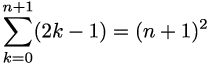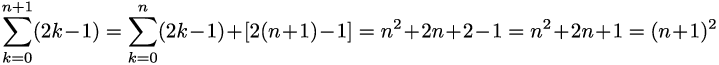# Math something

Few years ago I found an interesting pattern, playing with some programming algorithm. I am not sure if I defined the pattern well in terms of math language, despite I was trying to be as close as possible. The hypothesis is:

A sum of all members of consecutive natural odd numbers set equals to amount of set members squared.

Hence,

 Set Amount of set members Sum of set members Formula 1 1 1 1 = 1 ^ 1 1,3 2 4 1 + 3 = 2 ^ 2 1,3,5 3 9 1 + 3 + 5 = 3 ^ 2 1,3,5,7 4 16 1 + 3 + 5 + 7 = 4 ^ 2 1,3,5,7,9 5 25 1 + 3 + 5 + 7 + 9 = 5 ^ 2

Let’s prove the pattern works for any set by mathematical induction.## Proof

For n = 1 is true:Suppose that:We need to show that:Indeed,What proves the Hypothesis. I am totally sure the thing proven is something simple and very fundamental, but I still hope, I’m the first who discovered it:) I also hope there is practical application for it. Unfortunately, I’m mathematically ignorant and I have no clue on what the above means.